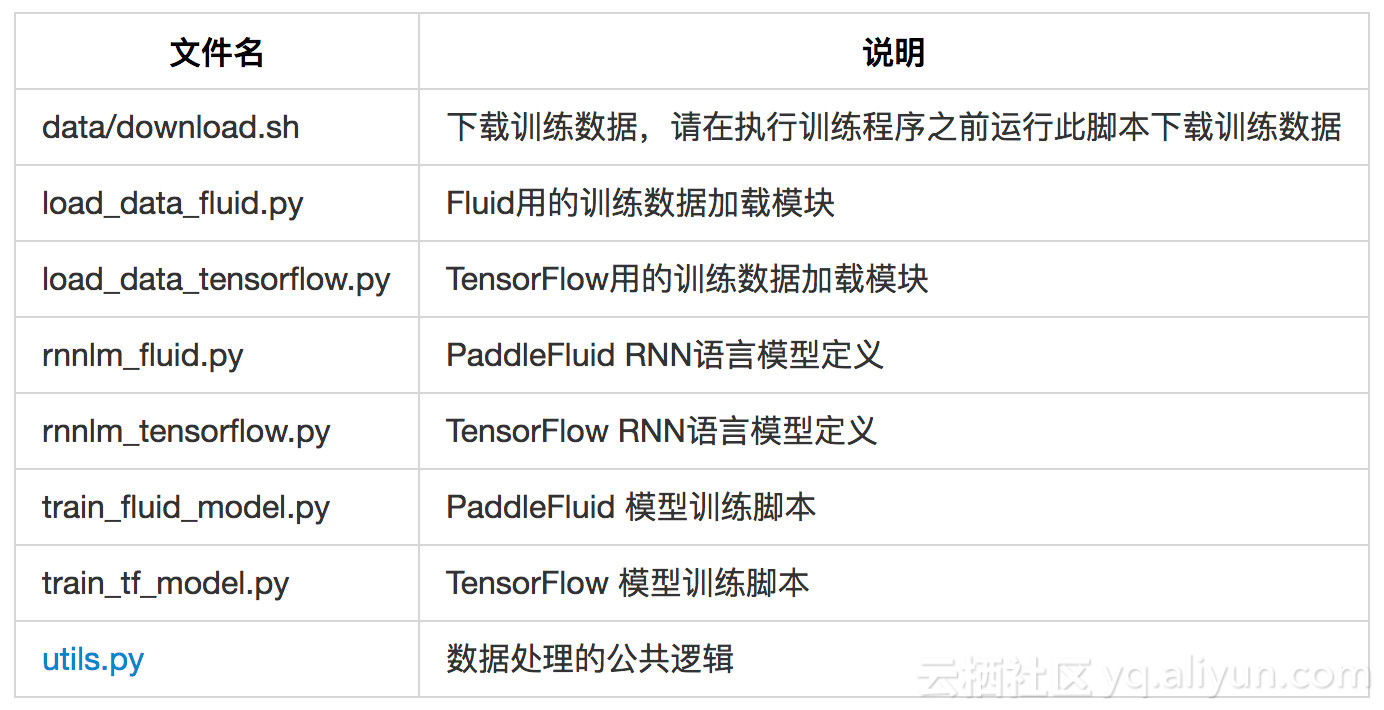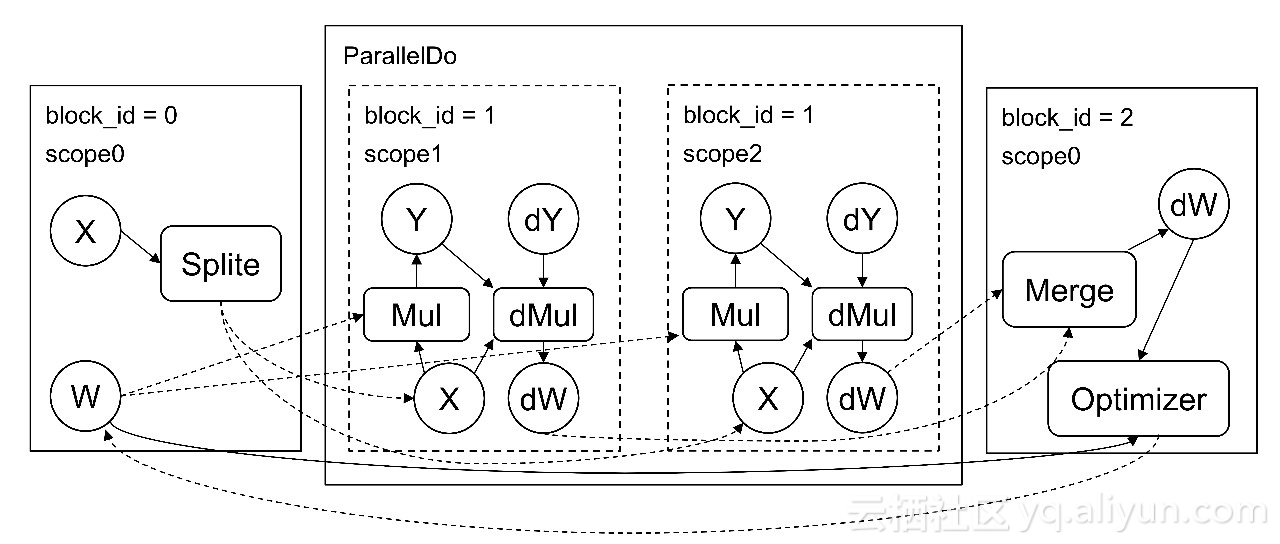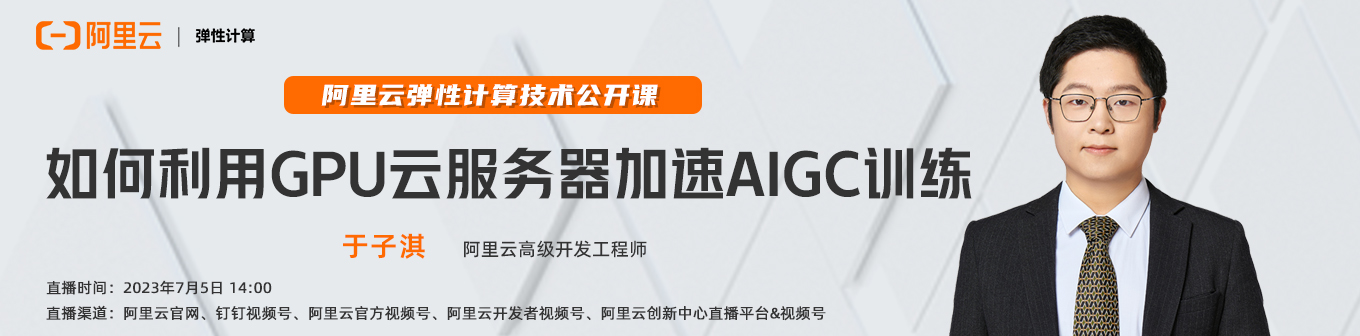+关注继续查看

1. 为 PaddleFluid 和 TensorFlow 模型添加上多 GPU 卡运行的支持。
2. 使用 TensorFlow 的 dataset API 为 TensorFlow 的 RNN 语言模型重写数据读取 部分，以提高 I/O 效率。sh download.sh


python train_fluid_model.py


python train_tf_model.py


| 1. 将模型参数拷贝到不同的设备
| 2. 对输入数据均匀切分到不同的计算设备
|||| 3. 多个设备并行进行前向计算
|||| 4. 多个设备形象进行反向计算
| 5. 多个设备计算的梯度在主卡合并
| 6. 计算参数更新量，更新参数
| to 1places = fluid.layers.get_places()
pd = fluid.layers.ParallelDo(places)

with pd.do():

prediction, cost = self.__network(word_, lbl_)

pd.write_output(cost)
pd.write_output(prediction)

cost, prediction = pd()
avg_cost = fluid.layers.mean(x=cost)


pd = fluid.layers.ParallelDo(places) 指定将在 那些设备上并行地执行。

parallel_do 会构建一段 context，在其中定义要并行执行的计算，调用 pd.read_input 切分输入数据，在 parallel_do 的 context 之外调用 pd() 获取合并后的最终计算结果。

with pd.do():
x_ = pd.read_input(x) # 切分输入数据 x
y_ = pd.read_input(y) # 切分输入数据 y

# 定义网络
cost = network(x_, y_)
pd.write_output(cost)
cost = pd() # 获取合并后的计算结果

TensorFlow中使用多GPU卡进行训练

TensorFlow 中实现多卡数据并行有多种方法，常用的包括单机 ParameterServer 模式；Tower 模式 ，甚至也 可以使用最新的 nccl  all reduce 系列 op 来实现梯度的聚合。这里我们以 Tower 模式为基础，介绍一种简单易用的多 GPU 上的数据并行方式。下面是核心代码片段，完整代码请参考 rnnlm_tensorflow.py。

def make_parallel(fn, num_gpus, **kwargs):
in_splits = {}
for k, v in kwargs.items():
in_splits[k] = tf.split(v, num_gpus)

out_split = []
for i in range(num_gpus):
with tf.device(tf.DeviceSpec(device_type="GPU", device_index=i)):
with tf.variable_scope(
tf.get_variable_scope(), reuse=tf.AUTO_REUSE):
out_i = fn(**{k: v[i] for k, v in in_splits.items()})
out_split.append(out_i)

self.batch_size)


make_parallel 的第一个参数是一个函数，也就是我们自己定义的如何创建神经网络模型函数。第二个参数指定 GPU 卡数，数据将被平均地分配给这些 GPU。除此之外的参数将以 keyword argument 的形式传入，是神经网络的输入层 Tensor 。

make_parallel 中使用 tf.split op 对输入数据 Tensor 进行切分，使用 tf.add_n 合并多个 GPU 卡上的计算结果。

self.cost = self.make_parallel(
self.build_model,
len(get_available_gpus()),
curwd=curwd,
nxtwd=nxtwd,
seq_len=seq_len)|
3天前
|
XML 数据格式 异构计算

12 1
|
18天前
|

41 0
|
2月前
|

|
3月前
|

552 0
|
5月前
|

PyTorch 中的多 GPU 训练和梯度累积作为替代方案
PyTorch 中的多 GPU 训练和梯度累积作为替代方案
65 0
|
5月前
|

37079 10|
5月前
|

gpu是什么和cpu的区别 模型训练
gpu是什么和cpu的区别 模型训练
460 1
|
6月前
|

635 39
|
6月前
|

3730 0
|
7月前
|

ViT系列 | 24小时用1张GPU训练一个Vision Transformer可还好？
ViT系列 | 24小时用1张GPU训练一个Vision Transformer可还好？
298 0